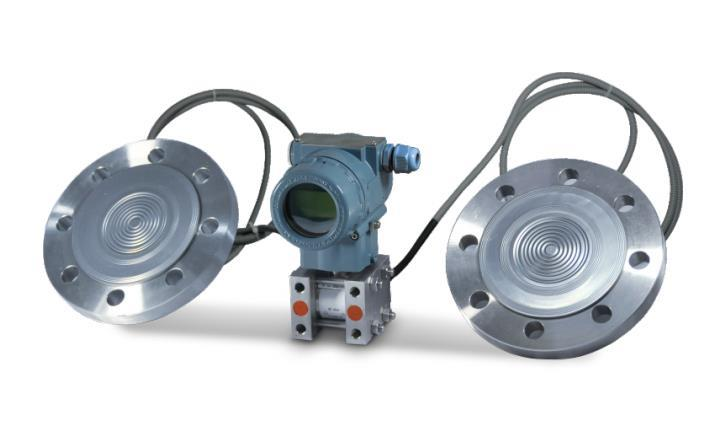﻿ 双法兰差压变送器的在液位测量中的调校方法与经验分享_淮安润中仪表科技有限公司

# 双法兰差压变送器的在液位测量中的调校方法与经验分享1、双法兰差压变送器迁移量、量程计算依据2、计算实例

①液位的zui大测量范围为△Pmax=H×ρ×g=2800×0.9×9.81=24.7 (kPa)
②当液位高度为Hmax时，双法兰差压变送器正压室所受的压力P+为P+=P0+H×ρ×g-h1×ρ0×g=2800×0.9×9.81-1200×1.0×9.81=12.949(kPa)
③双法兰差压变送器负压室所受的压力P-：P-=P0+(h-h1)×ρ0×g=(3800-1200)×1.0×9.81=25.506(kPa)
④双法兰差压变送器的zui大差压值△P：△P=P+-P-=H×ρ×g-h×ρ0×g=2800×0.9×9.81-3800×1.0×9.81=-12.557 (kPa)，此时变送器输出20mA，这个值作为双法兰差压变送器量程上限值
⑤双法兰差压变送器的负迁移量S负迁移：S负迁移=-(h×ρ0×g)=3800×1.0×9.81=-37.278kPa，此时变送器输出4mA，这个值作为双法兰差压变送器量程下限值